# Galois correspondence

(diff) ← Older revision | Latest revision (diff) | Newer revision → (diff)

between two partially ordered setsand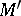A pair of mappingsand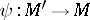which satisfy the following conditions:

if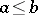, then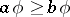;

if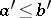, then;and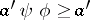.

Here,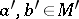.

The concept of a Galois correspondence is closely connected with the concept of closure in a partially ordered set; this means that if a Galois correspondence is established betweenand, the equalities,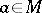, and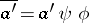,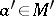, define closure operations (cf. Closure relation) inand, respectively. The concept of a Galois correspondence originated in Galois theory, which deals with the Galois correspondence between all intermediate subfields of an extensionand the system of subgroups of the Galois group of this extension.

How to Cite This Entry:
Galois correspondence. Encyclopedia of Mathematics. URL: http://encyclopediaofmath.org/index.php?title=Galois_correspondence&oldid=12061
This article was adapted from an original article by O.A. Ivanova (originator), which appeared in Encyclopedia of Mathematics - ISBN 1402006098. See original article